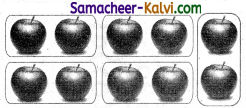## Samacheer Kalvi 3rd Standard Maths Book Solutions Term 3 Chapter 2 Numbers

Equal sharing and repeated subtraction (Text Book Page No. 11):

Question 1.
Suppose Kabilan has to share the mangoes among 15 of his friends. How many mangoes would each of them get? How Many steps would he require to share the mangoes among them?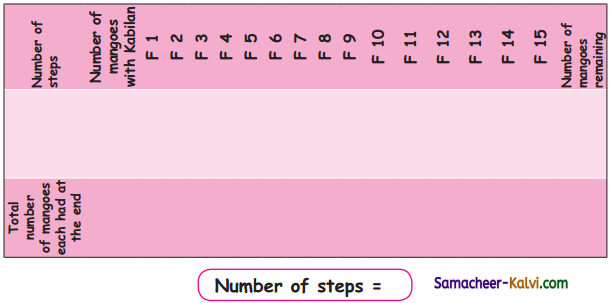Complete the number line.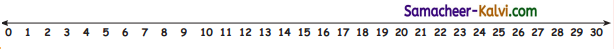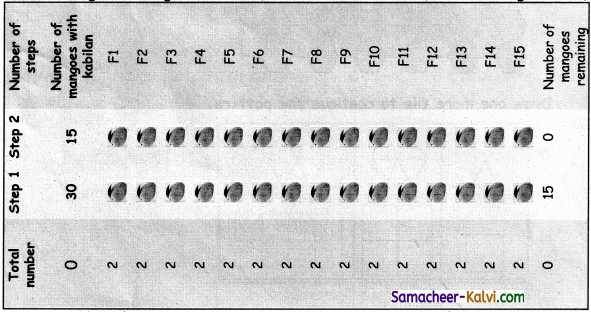Number of steps = 2

Complete the number line – 15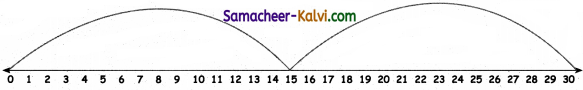Repeated subtraction statement 30 -15 -15 = 0
Kabilan shared 30 mangoes among 15 of his friends in 2 steps.
Each friend got 2 mangoes.Complete the table. (Text Book Page No. 12):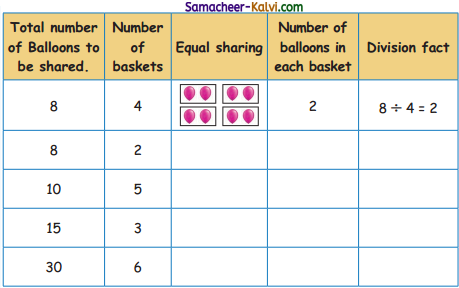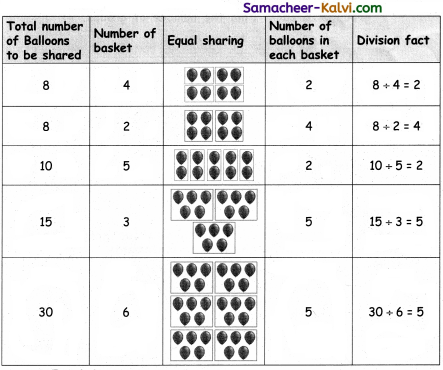Equal Sharing (Text Book Page No. 13):

Question 2.
Rangamma has 36 coconuts and arranges them into group of 4 each. Find the number of groups.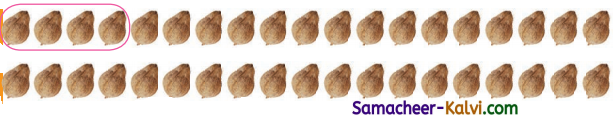The number line can be drawn as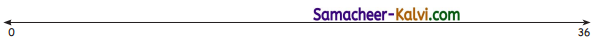The number statement is ___________.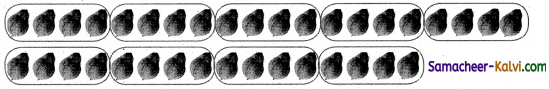The number line can be drawn as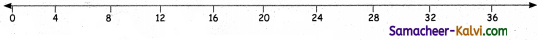The number statement is 36 ÷ 4 = 9.Question 3.
Rangamma has got 48 gooseberries and arranges them into group of 6 each. Find the number of groups.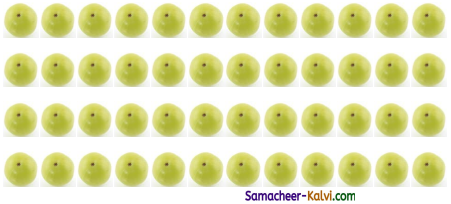The number line can be drawn as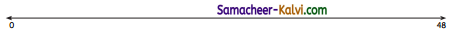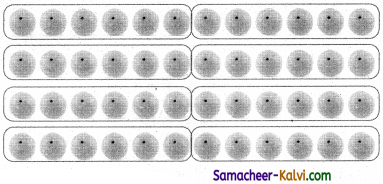The number can be drawn as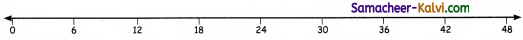The number statement is 48 ÷ 6 = 8.Question 4.
Find few other ways that Rangamma can group these 48 gooseberries and write the number statements.

a.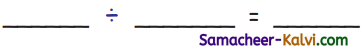48 ÷ 2 = 24

b.48 ÷ 3 = 16

c.48 ÷ 4 = 12d.48 ÷ 6 = 8

e.48 ÷ 8 = 6

f.48 ÷ 12 = 4g.48 ÷ 16 = 3

h.48 ÷ 24 = 2

i.48 ÷ 1 = 48Exercise (Text Book Page No. 14):

a. Divide 8 Balls into a group of 2 Balls each.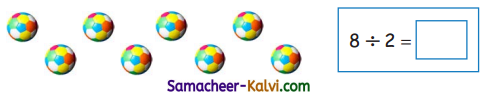8 ÷ 2 = 4b. Divide 15 oranges into a group of 3 each.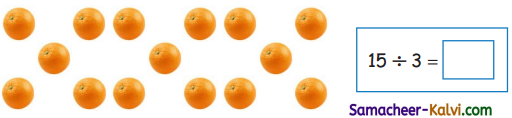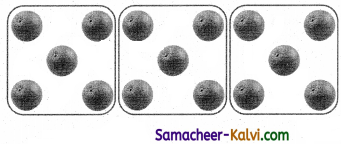15 ÷ 3 = 5c. Divide 20 cups into groups of 5 each.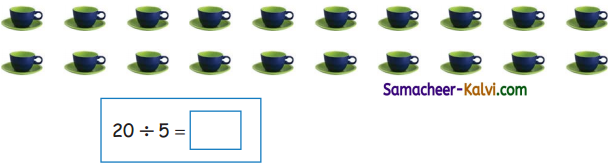20 ÷ 5 = 4Multiplication and Division (Text Book Page No. 17):

Question 1.
Construct the division fact for the multiplication tables 3.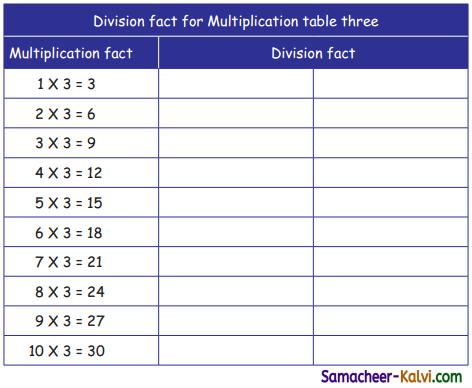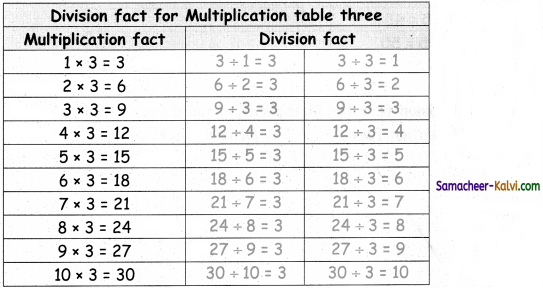Question 2.
Construct the division fact for the multiplication tables 4.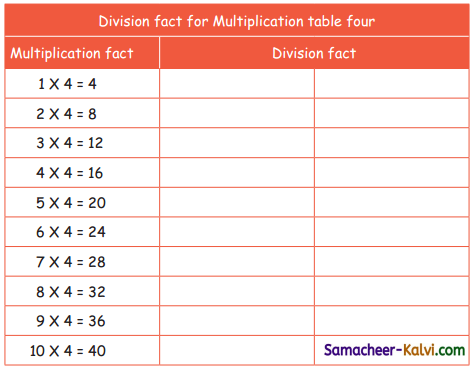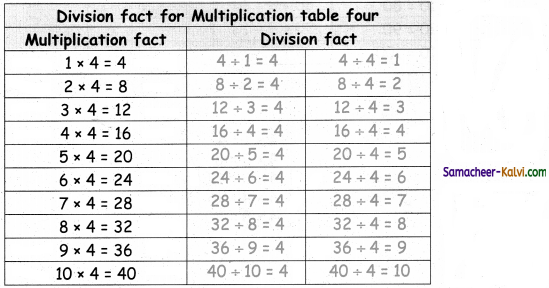Question 3.
Construct the division fact for the multiplication tables 5.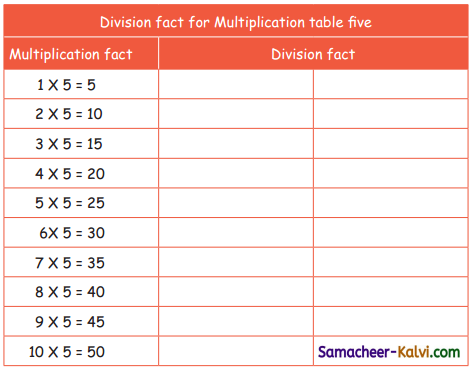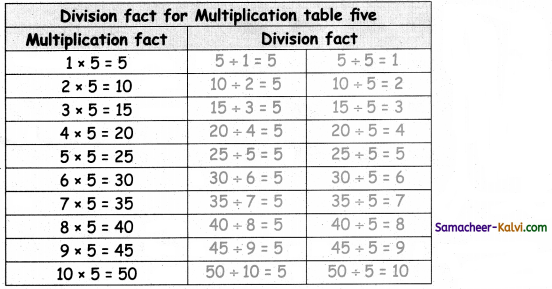Question 4.
Construct the division fact for the multiplication tables 10.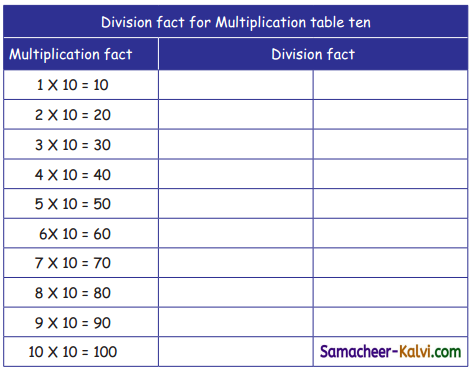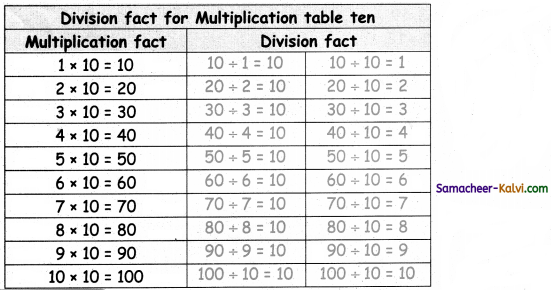Exercise (Text Book Page No. 19):

Divide and find the quotient.

Question 1.
20 ÷ 4 =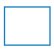4 × 1 = 4
4 × 2 = 8
4 × 3 = 12
4 × 4 = 16
4 × 5 = 20

20 ÷ 4 = 5

Question 2.
10 ÷ 2 =2 × 1 = 2
2 × 2 = 4
2 × 3 = 6
2 × 4 = 8
2 × 5 = 10

10 ÷ 2 = 5Question 3.
24 ÷ 3 =3 × 1 = 3
3 × 2 = 6
3 × 3 =9
3 × 4 = 12
3 × 5 = 15
3 × 6 = 18
3 × 7 = 21
3 × 8 = 24

24 ÷ 3 = 8

Question 4.
10 ÷ 10 =10 × 1 =10

10 ÷ 10 = 1Question 5.
30 ÷ 5 =5 × 1 = 5
5 × 2 = 10
5 × 3 = 15
5 × 4 = 20
5 × 5 = 25
5 × 6 = 30

30 ÷ 5 = 6

Question 6.
14 ÷ 2 =2 × 1 = 2
2 × 2 = 4
2 × 3 = 6
2 × 4 = 8
2 × 5 = 10
2 × 6 = 12
2 × 7 = 14

14 ÷ 2 = 7Question 1.
Divide 15 pencils into a group of 5 each.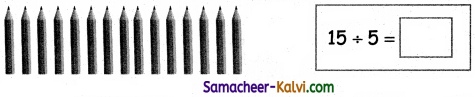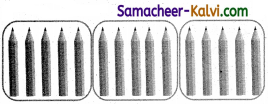15 ÷ 5 = 3Question 2.
Divide 16 balloons into groups of 4 each.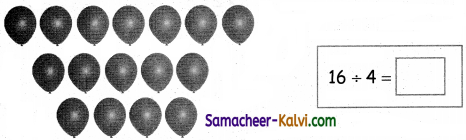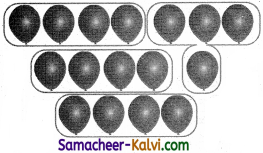16 ÷ 4 = 4Question 3.
Divide and find the quotient.

(i) 32 ÷ 4 =4 × 1 = 4
4 × 2 = 8
4 × 3 = 12
4 × 4 = 16
4 × 5 = 20
4 × 6 = 24
4 × 7 = 28
4 × 8 = 32

32 ÷ 4 = 8

(ii) 21 ÷ 3 =3 × 1 = 3
3 × 2 = 6
3 × 3 = 9
3 × 4 = 12
3 × 5 = 15
3 × 6 = 18
3 × 7 =21

21 ÷ 3 = 7Question 4.
Arjun has 10 apples. He divides them among 2 of his friends. How many apples would each friend get?